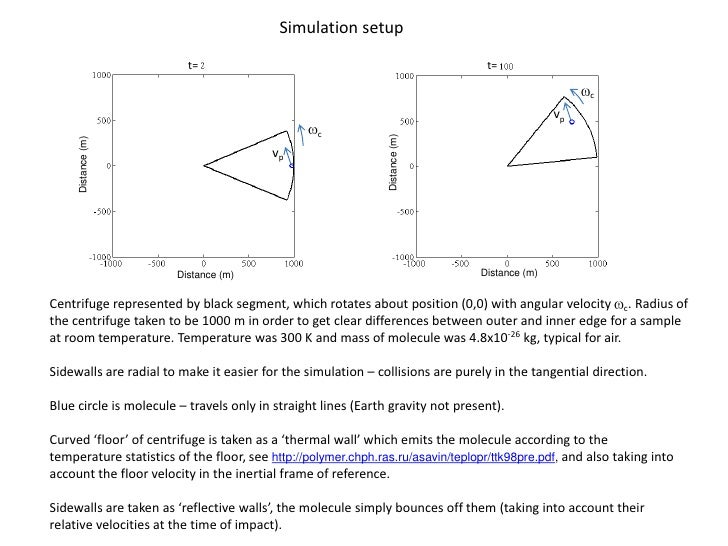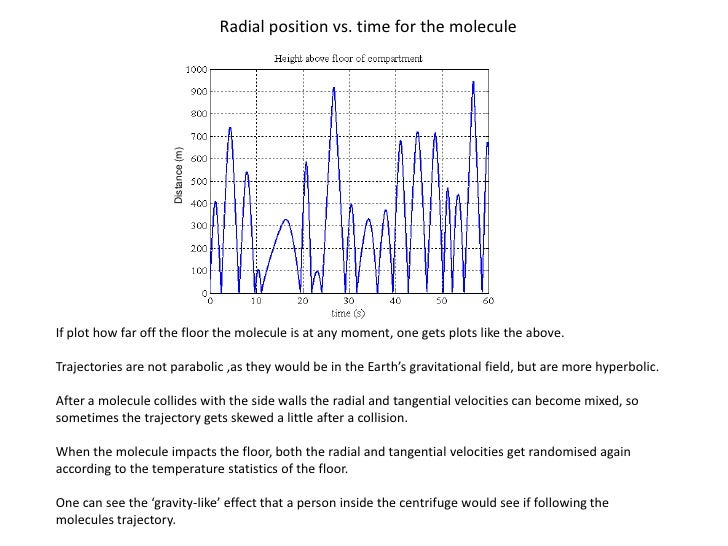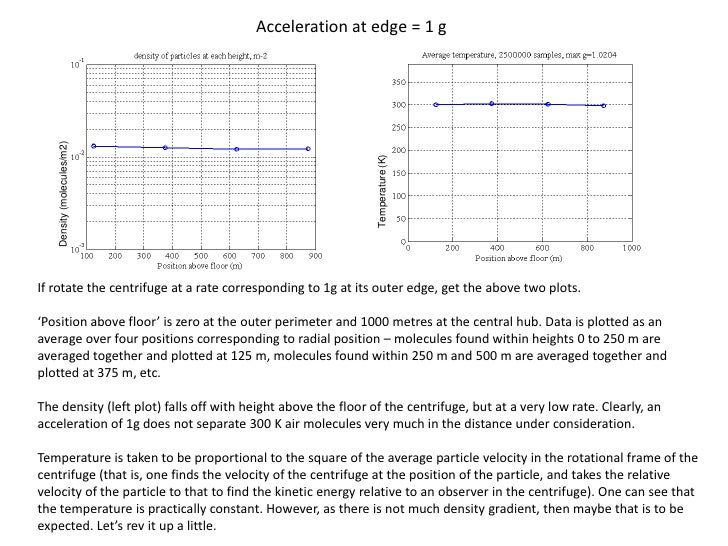Successfully reported this slideshow.Upcoming SlideShare
×

ofUpcoming SlideShare
Centrifugation
Next

0 Likes

Share

# Temperature gradient in a centrifuge

A molecule's trajectory is followed in a centrifuge. Each time the molecule hits the floor of the centrifuge it is given a random velocity according to the temperature of the floor. When it hits the sides of the centrifuge it simply reflects. By taking the average velocity of the particle versus position, a temperature profile can be calculated. It is found that the temperature profile is isothermal.

See all

### Related Audiobooks

#### Free with a 30 day trial from Scribd

See all
• Be the first to like this

### Temperature gradient in a centrifuge

1. 1. Simulation setup t= t= c vp c Distance (m) Distance (m) vp Distance (m) Distance (m) Centrifuge represented by black segment, which rotates about position (0,0) with angular velocity c. Radius of the centrifuge taken to be 1000 m in order to get clear differences between outer and inner edge for a sample at room temperature. Temperature was 300 K and mass of molecule was 4.8x10-26 kg, typical for air. Sidewalls are radial to make it easier for the simulation – collisions are purely in the tangential direction. Blue circle is molecule – travels only in straight lines (Earth gravity not present). Curved ‘floor’ of centrifuge is taken as a ‘thermal wall’ which emits the molecule according to the temperature statistics of the floor, see http://polymer.chph.ras.ru/asavin/teplopr/ttk98pre.pdf, and also taking into account the floor velocity in the inertial frame of reference. Sidewalls are taken as ‘reflective walls’, the molecule simply bounces off them (taking into account their relative velocities at the time of impact).
2. 2. Radial position vs. time for the molecule Distance (m) If plot how far off the floor the molecule is at any moment, one gets plots like the above. Trajectories are not parabolic ,as they would be in the Earth’s gravitational field, but are more hyperbolic. After a molecule collides with the side walls the radial and tangential velocities can become mixed, so sometimes the trajectory gets skewed a little after a collision. When the molecule impacts the floor, both the radial and tangential velocities get randomised again according to the temperature statistics of the floor. One can see the ‘gravity-like’ effect that a person inside the centrifuge would see if following the molecules trajectory.
3. 3. Acceleration at edge = 1 g Density (molecules/m2) Temperature (K) If rotate the centrifuge at a rate corresponding to 1g at its outer edge, get the above two plots. ‘Position above floor’ is zero at the outer perimeter and 1000 metres at the central hub. Data is plotted as an average over four positions corresponding to radial position – molecules found within heights 0 to 250 m are averaged together and plotted at 125 m, molecules found within 250 m and 500 m are averaged together and plotted at 375 m, etc. The density (left plot) falls off with height above the floor of the centrifuge, but at a very low rate. Clearly, an acceleration of 1g does not separate 300 K air molecules very much in the distance under consideration. Temperature is taken to be proportional to the square of the average particle velocity in the rotational frame of the centrifuge (that is, one finds the velocity of the centrifuge at the position of the particle, and takes the relative velocity of the particle to that to find the kinetic energy relative to an observer in the centrifuge). One can see that the temperature is practically constant. However, as there is not much density gradient, then maybe that is to be expected. Let’s rev it up a little.
4. 4. Acceleration at edge = 100 g Density (molecules/m2) Temperature (K) At higher ‘g’ values the density drops off faster, as expected. In this case the average density in the innermost portion of the centrifuge is about x100 less than in the outer portion. The temperature plot is still practically zero for the first three points. The rightmost point is down a bit, but this has run-to-run variation which is hard to stabilise. The reason is that because the ‘g’ factor of the centrifuge goes to zero at the hub, particles can contribute to the ensemble in disproportionate ways unless very large data sets are run to average these out. Also note that the third point is very slightly above average, even though the density is similar to the fourth point, so again one should not infer that there is a trend to the data.
5. 5. Mass separation at 100 g Density (molecules/m2) Here the temperature and speed of the centrifuge are kept constant, but the mass of the molecule is changed. The blue curve is for a molecule with mass = 2.4x10-26 kg, The red curve is for a molecule with mass = 4.8x10-26 kg. So one can see that a centrifuge can be used to separate molecules of different mass, as is well known.
6. 6. Summary A simulation was written for a particle in a centrifuge Floor of centrifuge taken to be a thermal wall Sidewalls of centrifuge taken to be reflective walls Even with a centrifuge 1000 m in radius and 100 g acceleration, there is no clear drop in temperature with radius Density drops off with radius, but at a rate less than a single exponential to be expected, as the ‘g’ factor decreases towards the centre of the centrifuge density drop-off is steeper at faster rotation rates, as expected. density drop-off proportional to mass as expected. At higher ‘g’ rates, the simulation goes a bit crazy a vacuum is formed in the inner radius of the centrifuge and the numerical statistics are not good need to run for a very long time to get a good sample of the velocity distributions. However, density factors of 100 do not give gradients, so no reason to expect they should start.

Total views

1,100

On Slideshare

0

From embeds

0

Number of embeds

1

14

Shares

0